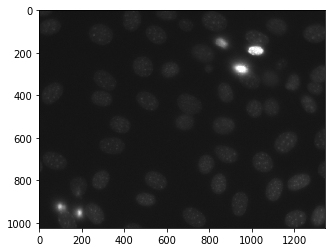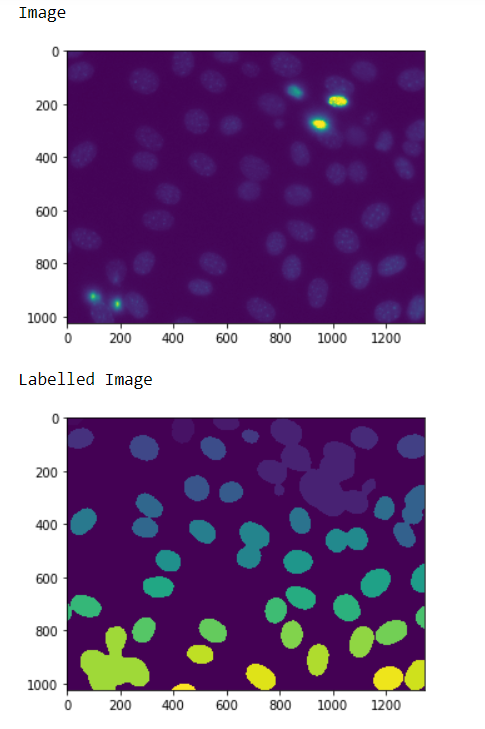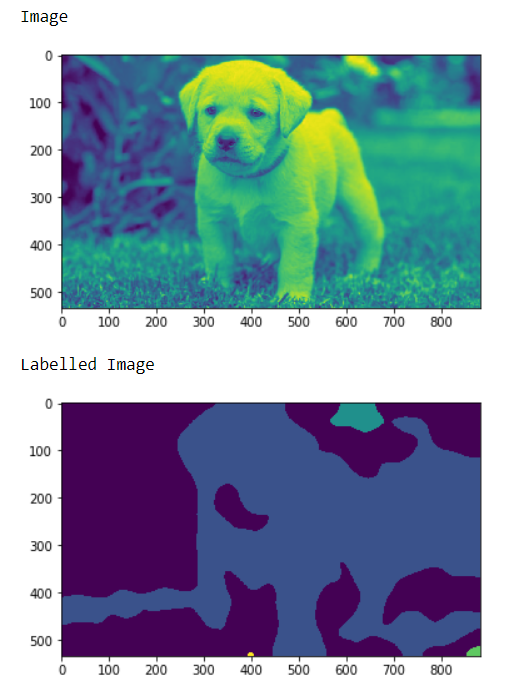GeeksforGeeks App
Open AppBrowser
Continue

# Mahotas – Labelled Image from the Normal Image

In this article we will see how we can create a labelled image from the normal image in mahotas. For this we are going to use the fluorescent microscopy image from a nuclear segmentation benchmark. We can get the image with the help of command given below

`mahotas.demos.nuclear_image()`

Below is the nuclear_imageLabelled images are integer images where the values correspond to different regions. I.e., region 1 is all of the pixels which have value 1, region two is the pixels with value 2, and so on
In order to do this we will use mahotas.label method

Syntax : mahotas.label(image)
Argument : It takes loaded image object as argument
Return : It returns the labelled image and the integer i.e number of labels

Note : The input of the label should be the filtered image object and it should have the threshold and it is preferred that image should have gaussian filter for removing sharper edges.
In order to filter the image we will take the image object which is numpy.ndarray and filter it with the help of indexing, below is the command to do this

`image = image[:, :, 0]`

Example 1 :

## Python3

 `# importing required libraries``import` `mahotas``import` `numpy as np``from` `pylab ``import` `imshow, show``import` `os` `# loading nuclear image``f ``=` `mahotas.demos.load(``'nuclear'``)` `# setting filter to the image``f ``=` `f[:, :, ``0``]` `# show the image``print``(``"Image"``)``imshow(f)``show()` `# setting gaussian filter``f ``=` `mahotas.gaussian_filter(f, ``4``)` `# setting threshold value``f ``=` `(f> f.mean())` `# creating a labelled image``labelled, n_nucleus ``=` `mahotas.label(f)` `# showing the labelled image``print``(``"Labelled Image"``)``imshow(labelled)``show()`

Output :Example 2 :

## Python3

 `# importing required libraries``import` `numpy as np``import` `mahotas``from` `pylab ``import` `imshow, show` `# loading image``img ``=` `mahotas.imread(``'dog_image.png'``)``  ` `# filtering the image``img ``=` `img[:, :, ``0``]``   ` `print``(``"Image"``)``# showing the image``imshow(img)``show()`` ` `# setting gaussian filter``gaussian ``=` `mahotas.gaussian_filter(img, ``15``)` `# setting threshold value``gaussian ``=` `(gaussian > gaussian.mean())` `# creating a labelled image``labelled, n_nucleus ``=` `mh.label(gaussian)`` ` `print``(``"Labelled Image"``)``# showing the gaussian filter``imshow(labelled)``show()`

Output :My Personal Notes arrow_drop_up